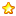IP: 44.197.101.251

##用戶名 Email 自動登錄 找回密碼 密碼 立即註冊
 搜索 熱搜: 活動 交友 discuz

# [配裝配點] 整理為簡單的+20最終評鑑2主題 43積分43發表於 2023-10-27 02:41:32 | 顯示全部樓層 |閱讀模式
 以下為武器部分(包含影子裝及神武) 精煉+20，評鑑等級D： 等於額外增加ATK/MATK = 20(精煉值)x1(評鑑等級)x1(評鑑等級)=20 等於額外增加CRI= 20(精煉值)x1(評鑑等級)x1(評鑑等級)x0.5=10 精煉+20，評鑑等級C： 等於額外增加ATK/MATK = 20(精煉值)x2(評鑑等級)x2(評鑑等級)=80 等於額外增加CRI = 20(精煉值)x2(評鑑等級)x2(評鑑等級)x0.5=40 精煉+20，評鑑等級B： 等於額外增加ATK/MATK = 20(精煉值)x3(評鑑等級)x3(評鑑等級)=180 等於額外增加CRI= 20(精煉值)x3(評鑑等級)x3(評鑑等級)x0.5=90 精煉+20，評鑑等級A： 等於額外增加ATK/MATK = 20(精煉值)x4(評鑑等級)x4(評鑑等級)=320 等於額外增加CRI= 20(精煉值)x4(評鑑等級)x4(評鑑等級)x0.5=160 以下為裝備部分(包含影子裝及神武) 精煉+20，評鑑等級D： 等於額外增加DEF/MDEF = 20(精煉值)x1(評鑑等級)x1(評鑑等級)x 0.5=10 等於額外增加HP =1000x20(精煉值)x1(評鑑等級)=20000 精煉+20，評鑑等級C： 等於額外增加DEF/MDEF = 20(精煉值)x2(評鑑等級)x2(評鑑等級)x 0.5=40 等於額外增加HP =1000x20(精煉值)x2(評鑑等級)=40000 精煉+20，評鑑等級B： 等於額外增加DEF/MDEF = 20(精煉值)x3(評鑑等級)x3(評鑑等級)x 0.5=90 等於額外增加HP =1000x20(精煉值)x3(評鑑等級)=60000 精煉+20，評鑑等級A： 等於額外增加DEF/MDEF = 20(精煉值)x4(評鑑等級)x4(評鑑等級)x 0.5=160 等於額外增加HP =1000x20(精煉值)x4(評鑑等級)=80000
 您需要登錄後才可以回帖 登錄 | 立即註冊 本版積分規則 回帖後跳轉到最後一頁

Archiver|小黑屋|無管地帶IP: 44.197.101.251

GMT+8, 2023-12-5 02:32 , Processed in 0.098587 second(s), 22 queries .